﻿ 20-sim webhelp > Toolboxes > Control Toolbox > B-Spline Network Editor > Training a 1-D B-Spline Network

# Training a 1-D B-Spline Network

Navigation:  Toolboxes > Control Toolbox > B-Spline Network Editor >

# Training a 1-D B-Spline Network## Non Linear Function

In this lesson we will use a B-spline network with one input to approximate the simple non-linear function:

F = input2

The input, x , of this function ranges from 0 to 10. It will be approximated by a B-Spline network with one input. As is shown in the figure below (left), 10 first order B-splines are used. The tenth B-spline is indicate with fat lines. The output of the B-spline network is a combination of all ten B-Splines. In other words, the non-linear function will be approximated by 10 intervals of constant value.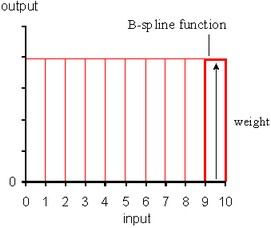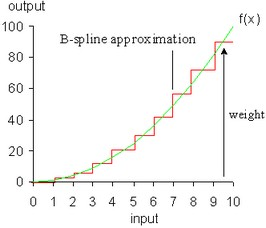At the start the B-spline has undefined weights. They can either be chosen at random values or all set to zero. A normal session should therefore start with training the network to adjust the weights to give a best approximation of the non-linear function. With these weights we can run the network with any desired input signal.

## Defining the B-Spline Network

We will use the model below to train the B-spline network. It has already been prepared for you.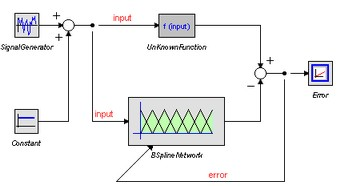1 In the Editor from the File menu select Open.
 2 In the model library choose Examples\Control\Neural Networks
 3 Select the model 1DBSplineNetwork-Train.emx. Now the predefined model will be opened.
 4 From the menu select Model and Start Simulator. Now a Simulator will be opened with the predefined experiment loaded.
 5 From Simulator menu click Simulation and Run. The results will look like: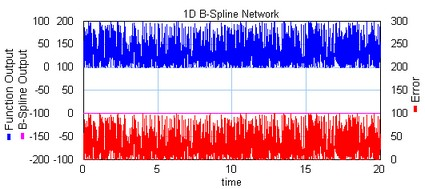As you can see, the B-Spline output is zero and there is no learning. Now we are going to change the settings of the B-Spline Network.

## Training the Network

 6 Return to the Editor. Select the BSplineNetwork model and click GoDown from the Model menu. Now the B-Spline Editor will pop-up. Change the settings (Network Order, Learning Rate, Input Upper Limit, Save Weights) until it looks like: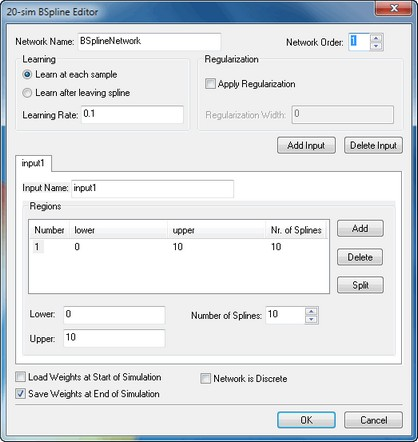7 Click OK to close the B-Spline Editor. Not that the icon of the BSplineNetwork model has changed to correspond with the first order splines that are used. From the Editor menu click Model and Check Complete Model. This will implement the changes in settings.
 8 Return to the Simulator and click Simulation and Run. The results will look like: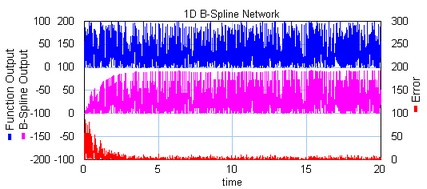As you can see the error rapidly descent, due to the network learning. After the Simulation Run, Save dialog opens, asking you to enter a name for the Weights File. This is a file that contains all the weights of the trained B-Spline network. We can use these weights for normal runs with the network.

 9 Enter file name (do not use the predefined weights file!), e.g. test.wgt and click Save.
 10 Close the Simulator.

Now all is prepared to perform a normal run with the Network.